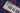/musictheorybook

# Modulo Arithmetic

What time is four hours later than 10 o'clock? When we make calculations like this, we are doing modular arithmetic . Modular arithmetic is…What time is four hours later than 10 o’clock?

When we make calculations like this, we are doing modular arithmetic. Modular arithmetic is like regular arithmetic, except that the numbers “wrap around” or restart when they reach a certain value, called the modulus. In the case of our 12-hour clock, the modulus is 12.

Musical structures can often be best understood using this modular arithmetic. Think of the C-major scale. We begin on C, then D, E, F, G and back to A before B and returning to C. This is a modular system — we might call it modulo-G because after G we go back to the beginning (A).

In post-tonal music, once we assume octave and enharmonic equivalence, our pitch-class environment includes twelve unique pitch classes, just like the twelve hours on the clock. In this universe, modular arithmetic is a very useful way to imagine getting around.

Counting in this modulo 12 (or mod12) universe works just as in basic math [“1, 2, 3, …”], but after 11, we “begin again” at 0. (Note that while clocks start at 1 and end on 12, modular arithmetic always (re)starts with zero.) Conversely, when counting down [“10, 9, 8, …”], we follow 0 with 11.

While we are used to thinking of numbers on an infinite line, modular thinking wraps them into a finite number, generally represented by a circle. On this circle, all values are a number from 0 to 11.

Pitch-Class SpaceTo add or subtract in mod12, perform the calculation in the usual manner (7 + 15 = 22) and then add or subtract 12s until you get a number from 0 to 11 (22 - 12 = 10).

Adding and subtracting can represent many musical ideas: moving seven half steps above D takes you to A (2 + 7 = 9); combining 2 half steps and 11 half steps produces 1 half step (2 + 11 = 1; or starting with C, moving up 2 half steps reaches D, and 11 more C-sharp — 1 higher than the original C).

Modular arithmetic is a quick way to calculate various intervals between pitches or pitch classess. Some examples:

What is the interval class from pitch class 7 (G) to pitch class 10 (B-flat)? 10 - 7 = 3 What is the pitch class 5 semitones above B-natural (11)? 11 + 5 = 4. That is, E.#### Joey Etamity

Software Engineer Discover the harmony of code💻 and music🎹.